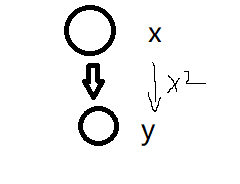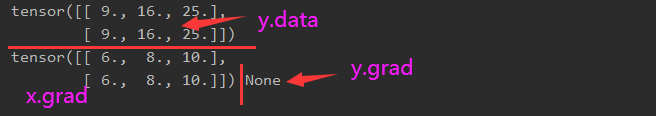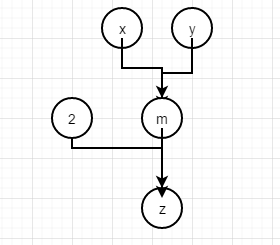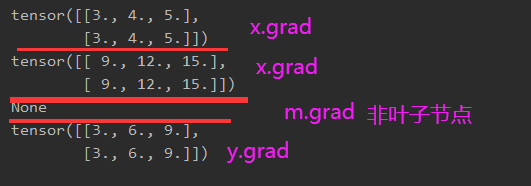2020-03-11 17:14:41

# https://www.cnblogs.com/henuliulei/p/11363121.htmlpytorch
更多相关内容
• 反向传递法则是深度学习中最为重要的一部分，torch中的backward...>>> from torch.autograd import Variable >>> x = Variable(torch.ones(2,2), requires_grad=True) >>> y = x + 2 >>> y.grad_fn Out: <torch> >

千次阅读 2021-08-03 15:26:13

PyTorch0.4.0之后Variable 已经被PyTroch弃用

使用Variable.data的效果和使用tensor.data一样

诸如Variable.backward()，Variable.detach()，Varialbe.register_hook()等方法直接移植到了tensor上，在tensor上可以直接使用同名的函数

此外，现在可以直接使用torch.randn()，torch.zeros()，torch.ones()等工厂方法直接用requires_gard=True属性生成tensor，例如

autograd_tensor = torch.randn((2, 3, 4), requires_grad=True)

展开全文pytorch
• Variable类和计算图，使用的参数 简单的建立一个计算图，便于理解几个相关知识点： requires_grad 是否要求导数，默认False，叶节点指定True后，依赖节点都被置为True .backward() 根Variable的方法会反向求解叶V

## 参考 的博客

1、https://blog.csdn.net/CSDN_of_ding/article/details/110691635
2、https://blog.csdn.net/e01528/article/details/84069243

## Variable类和计算图，使用的参数

简单的建立一个计算图，便于理解几个相关知识点：
.is_leaf 是否是叶节点

参数说明:
variables (variable 列表) – 被求微分的叶子节点 。
retain_variables (bool) – True,计算梯度时所需要的buffer在计算完梯度后不会被释放。如果想对一个子图多次求微分的话，需要设置为True。

## 个人程序实例1及结果

import torch
x = torch.Tensor([[3,4,5],[3,4,5]])
x = Variable(x, requires_grad = True)
y = x*x  # x^2
y.backward(torch.ones(x.size()))
print(y.data)## 个人程序实例2及结果

保留了上一次的结果，才能再一次backward（）否则图也解散第二次backward（）会报错：Trying to backward through the graph a second time, but the buffers have already been freed. Specify retain_graph=True when calling backward the first time

import torch
x = torch.Tensor([[3,4,5],[3,4,5]])
y = Variable(x, requires_grad = True)
x1 = torch.Tensor([[1,2,3],[1,2,3]])
x = Variable(x1, requires_grad = True)
m = x*y
# 这里retain_graph=True必须有，否则因为backward将图结构解散，下面再次的backward会报错
m.backward(torch.ones(x.size()),retain_graph=True)
z = m*2
# z.backward(torch.ones(x.size()),retain_graph=True)
z.backward(torch.ones(x.size()))可以求得x，y的梯度值，但不能求到m的梯度值展开全文• import numpy as np import torch import torch.nn datas = np.array([[1, 2], [3, 4]]) ...input = torch.autograd.Variable(torch.from_numpy(datas).float(), requires_grad=True) target = torch.autog.
import numpy as np
import torch
import torch.nn

datas = np.array([[1, 2], [3, 4]])
labels = np.array([[2, 3], [4, 5]])
loss_fn = torch.nn.MSELoss()

print(input)
print(target)

loss = loss_fn(input, target)
print(loss)

展开全文深度学习
• 一、了解Variable 顾名思义，Variable就是 变量 的意思。实质上也就是可以变化的量，区别于int变量，它是一种可以变化的变量，这正好就符合了反向传播，参数更新的属性。 具体来说，在pytorch中的Variable就是一个...
• 目录 神经网络 自动梯度 6.2.1　torch.autogradVariable 模型搭建和参数优化 6.3.1 PyTorch之torch.nn 6.3.2 PyTorch之torch.optim 神经网络 一个典型的神经网络的训练过程大致分为以下几个步骤： 1.首先定义神经...
•pytorch
• 直接把cuda（async=true）中的async=true去掉即可。pytorch python 机器学习
• 也就是所谓的 "Extending torch.autograd"。 Function与Module的差异与应用场景 Function与Module都可以对pytorch进行自定义拓展，使其满足网络的需求，但这两者还是有十分重要的不同： Function一般只定义一个...Pytorchpytorch 深度学习 人工智能pytorch 深度学习 人工智能
• torch.autograd提供实现任意标量值功能的自动区分的类和功能。 它需要对现有代码进行最小的更改，只需将所有张量包装在Variable对象中。 Variable API与常规Tensor API几乎相同（除了几个需要对梯度计算的输入进行...对象pytorch python
• 文章目录前言一、概述二、例程三、官方的...torch.autograd.Function 只需要实现两个 静态方法： forward可以有任意多个输入、任意多个输出，但是输入和输出必须是Variable。 backward的输入和输出的个数就是forwa.
• 文章目录1. Function理解2. Function与Module差异与应用场景3....回顾下VariableVariable就像是计算图中的节点，保存计算结果（包括前向传播的激活值，反向传播的梯度），而Function就像计算图中的边，实现Variablepytorchpytorch 人工智能 python
• Pytorch torch.autogradtorch.optim的区别//一个求模型里面的梯度，一个通过再梯度来更新模型参数权重 #更新权重值，更新过程使用下面的公式： weight = weight + learning_rate * gradient 1.Pytorch ...pytorch 深度学习 pythonpytorch 深度学习 机器学习pytorch
• 前言：pytorch的灵活性体现在它可以任意拓展我们所需要的内容，前面讲过的自定义模型、自定义层、自定义激活函数、自定义损失函数都属于pytorch的拓展，这里有三个重要的概念需要事先...包装torch普通函数和torch.nn......